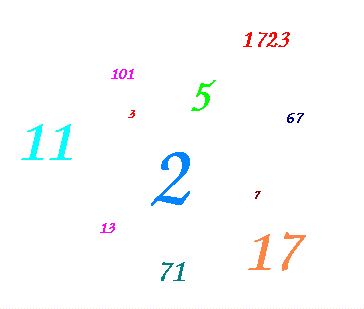# Prime NumbersMathematicians have a special fondness for definitions. The objects they study often have no physical presence: you can't touch them, weigh them, or smell them. So, precise definitions are used to provide a solid underpinning to their subject. Definitions are made for two reasons. Sometimes a mathematician makes a definition in order to have a simple shorthand term for a more complicated object that was needed to make sense of a proof. In other cases, a definition is a kind of generalization, made to unite lots of examples and special cases into a single kind of thing deserving further study. In the present document, we want to investigate some of the consequences of a single, simple definition of the latter kind.

Definition: An integer p is called a prime number if the only positive integers that divide p are 1 and p itself. Integers that are not prime are called composite.

Here are the first few prime numbers:

$2, 3, 5, 7, 11, 13, 17, 19, 23, 29, 31, ...$

Prime numbers are special because they are the elementary building blocks of the multiplicative structure on the integers; every integer can be written in only one way as a product of its prime factors. The mathematically precise version of this assertion is known as the The Fundamental Theorem of Arithmetic.

There are a number of interesting questions you can ask about prime numbers and factorizations.

Disclaimer: This page was last updated on 12 October 2002. It is entirely possible that the information contained herein no longer has any connection with reality (assuming it ever did). Feel free to send constructive comments or inane criticisms to:
Kevin R. Coombes
Department of Biostatistics
University of Texas M.D.Anderson Cancer Center
1515 Holcombe Blvd., Box 447
Houston, TX 77030